#Get Interactive Plots Directly With Pandas

Telling a story with data is a core function for any Data Scientist, and creating data visualizations that are simultaneously illuminating and appealing can be challenging. This tutorial reviews how to create Plotly and Bokeh plots directly through Pandas plotting syntax, which will help you convert static visualizations into interactive counterparts -- and take your analysis to the next level.

By Parul Pandey, Data Science at H2O.ai | Editor @wicds.Infographic vector created by macrovector — www.freepik.com.

Data exploration is by far one of the most important aspects of any data analysis task. The initial probing and preliminary checks that we perform, using the vast catalog of visualization tools, give us actionable insights into the nature of data. However, the choice of visualization tool at times is more complicated than the task itself. On the one hand, we have libraries that are easier to use but are not so helpful in showing complex relationships in data. Then there are others that render interactivity but have a considerable learning curve. Fortunately, some open-source libraries have been created that try to address this pain point effectively.

In this article, we’ll look at two such libraries, namely pandas_bokeh and cufflinks. We’ll learn how to create plotly and bokeh charts with the basic pandas plotting syntax, which we all are comfortable with. Since the article's emphasis is on the syntax rather than the types of plots, we’ll limit ourselves to the five basic charts, i.e., line charts, bar charts, histograms, scatter plots, and pie charts. We’ll create each of these charts first with pandas plotting library and then recreate them in plotly and bokeh, albeit with a twist.

### Import the Dataset

We’ll work with the NIFTY-50 dataset. The NIFTY 50 index is the National Stock Exchange of India’s benchmark for the Indian equity market. The dataset is openly available on Kaggle, but we’ll be using a subset of the data containing the stock value of only four sectors, i.e., bank, pharma, IT, and FMCG.

Let’s import the necessary libraries and dataset required for the visualization purpose:

```# Importing required modules
import pandas as pd
import numpy as np
import matplotlib.pyplot as plt
%matplotlib inline

```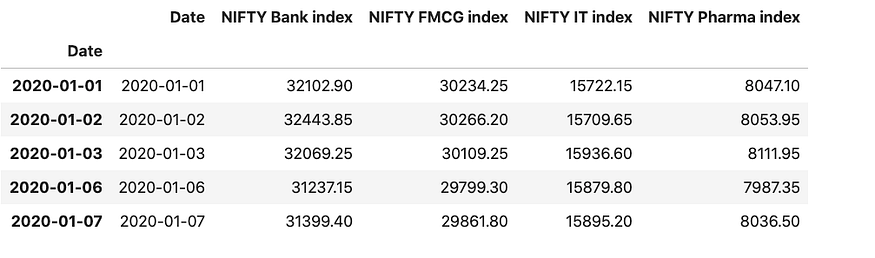Combined dataframe consisting of NIFTY indices of the bank, pharma, IT, and FMCG sectors.

We can also resample/aggregate the data by month-end. The pandas’ library has a resample() function, which resamples the time series data.

```nifty_data_resample = nifty_data.resample(rule = 'M').mean()
nifty_data_resample

```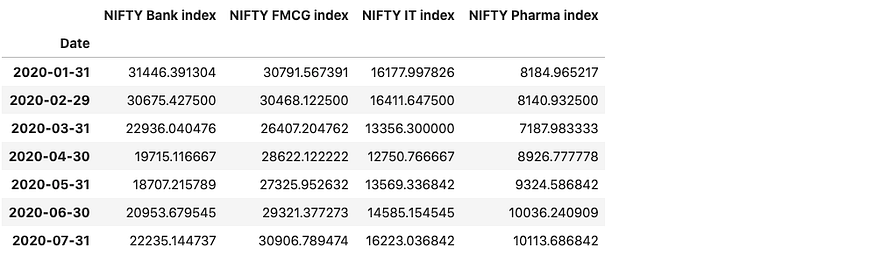Now that we have our dataframes ready, it is time to visualize them via different plots.

### Plotting with Pandas directly

Let’s begin with the most straightforward plotting technique — pandas’ plotting functions. To plot a graph using pandas, we’ll call the .plot() method on the dataframe.

Syntaxdataframe.plot()

The plot method is just a simple wrapper around matplotlib’s plt.plot()We can also specify some additional parameters like the ones mentioned below:

```Some of the important Parameters
--------------------------------

x : label or position, default None
Only used if data is a DataFrame.
y : label, position or list of label, positions, default None

title: title to be used for the plot

X and y label: Name to use for the label on the x-axis and y-axis.

figsize : specifies the size of the figure object.
kind : str
The kind of plot to produce:

- 'line' : line plot (default)
- 'bar' : vertical bar plot
- 'barh' : horizontal bar plot
- 'hist' : histogram
- 'box' : boxplot
- 'kde' : Kernel Density Estimation plot
- 'density' : same as 'kde'
- 'area' : area plot
- 'pie' : pie plot
- 'scatter' : scatter plot
- 'hexbin' : hexbin plot.

```

For a complete list of the parameters and their usage, please refer to the documentation. Let’s now look at ways to create different plots. In this article, we’ll not go into detail explaining each plot. We’ll only focus on the syntax, which is self-explanatory if you have some experience in pandas. For a detailed understanding of the pandas’ plots, this article will be helpful.

1. Line Plot
```nifty_data.plot(title='Nifty Index values in 2020',
xlabel = 'Values',
figsize=(10,6);

```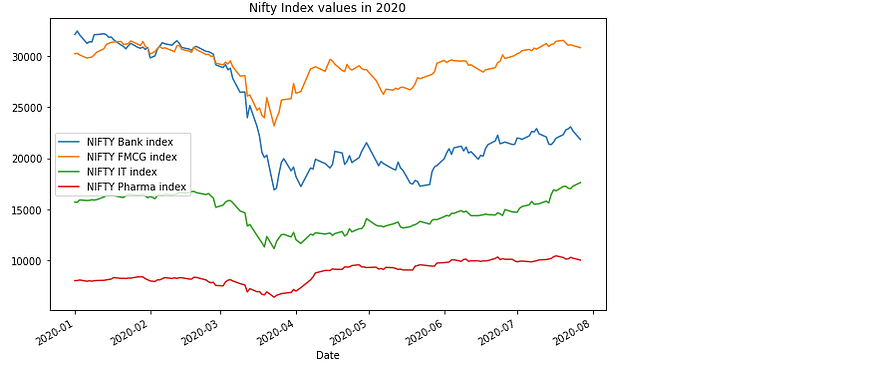Line plot with pandas plotting.

1. Scatter Plot
```nifty_data.plot(kind='scatter',
x='NIFTY FMCG index',
y='NIFTY Bank index',
title = 'Scatter Plot for NIFTY Index values in 2020',
figsize=(10,6));

```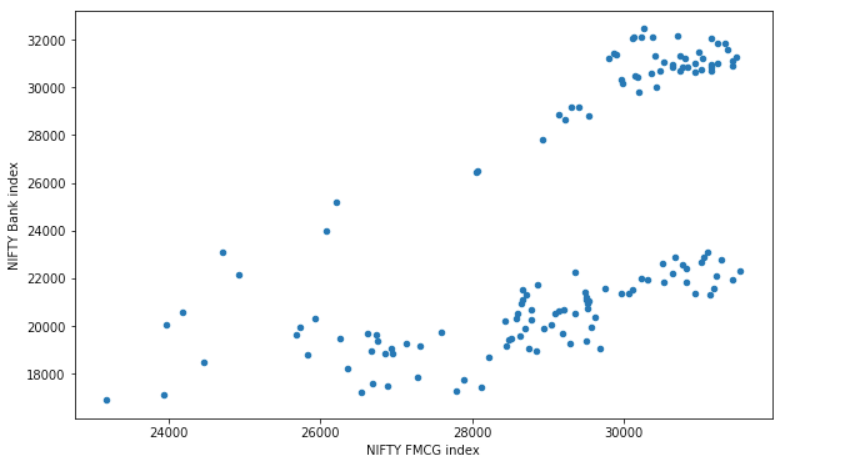Scatter plot with pandas plotting.

1. Histograms
```nifty_data[['NIFTY FMCG index','NIFTY Bank index']].plot(kind='hist',figsize=(9,6), bins=30);

```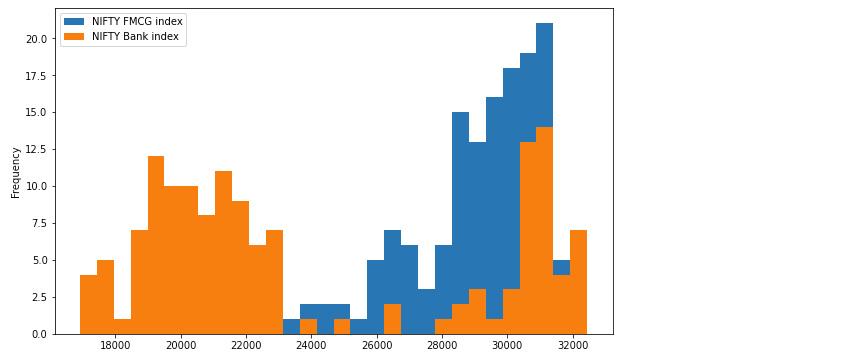Histogram with pandas plotting.

1. Bar plots
```nifty_data_resample.plot(kind='bar',figsize=(10,6));

```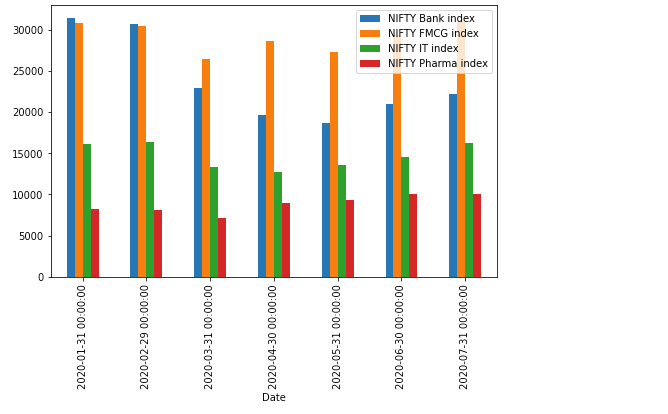Bar plot with pandas plotting.

• Stacked bar plots
```nifty_data_resample.plot(kind='barh',figsize=(10,6));

```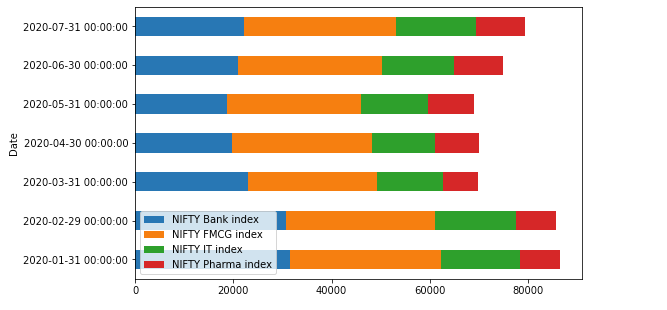Stacked Bar plot with pandas plotting.

1. Pie Charts
```nifty_data_resample.index=['Jan','Feb','March','Apr','May','June','July']
nifty_data_resample['NIFTY Bank index'].plot.pie(legend=False, figsize=(10,6),autopct='%.1f');

```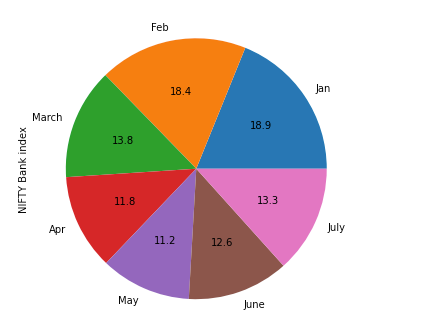Pie Chart with pandas plotting.

These were some of the charts that can be directly created with pandas’ dataframes. However, these charts lack interactivity and capabilities like zoom and pan. Let’s now change these existing charts in syntax into their fully interactive counterparts with just a slight change in the syntax.

### Bokeh Backend for Pandas — plotting with Pandas-Bokeh.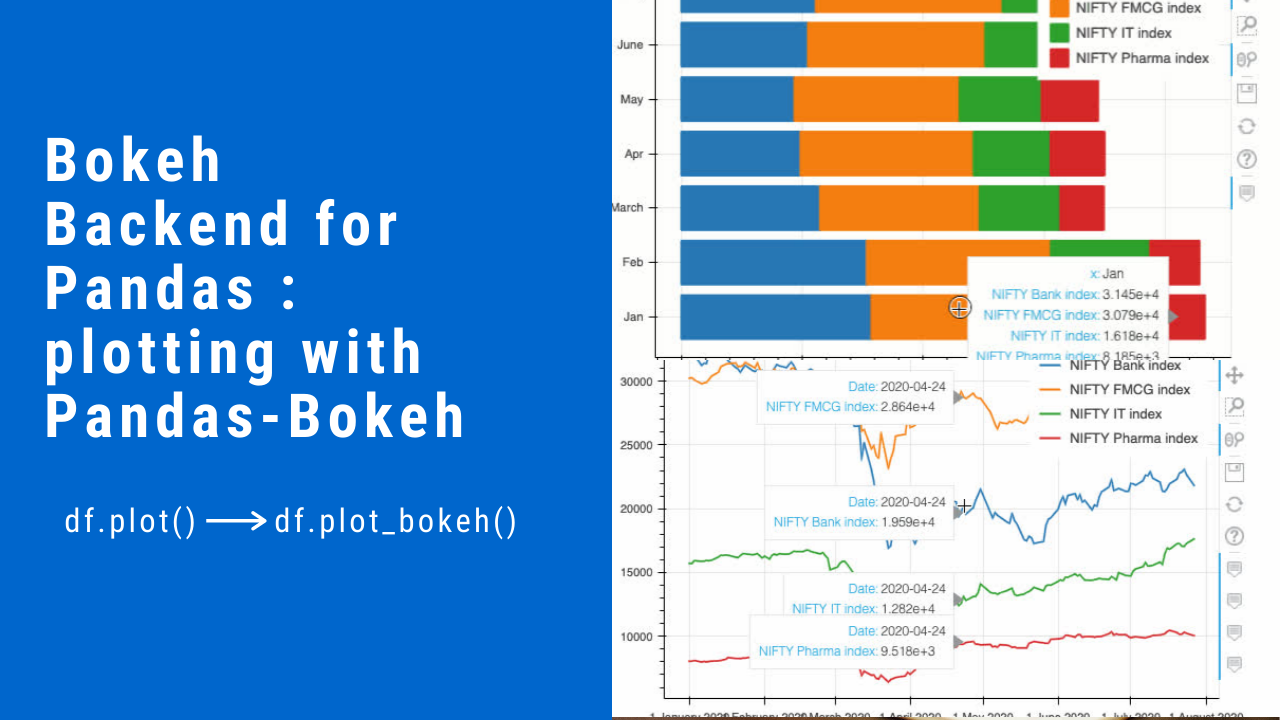Image by Author.

The bokeh library clearly stands out when it comes to data visualizationsThe Pandas-Bokeh provides a bokeh plotting backend for PandasGeoPandas, and Pyspark DataFrames. This backend adds a plot_bokeh() method to the DataFrames and Series.

Installation

Pandas-Bokeh can be installed from PyPI via pip or conda:

```pip install pandas-bokeh

or

conda install -c patrikhlobil pandas-bokeh

```

Usage

The Pandas-Bokeh library should be imported after Pandas, GeoPandas, or Pyspark.

```import pandas as pd
import pandas_bokeh

```

Then one needs to define the plotting output, which can be either of the two:

```pandas_bokeh.output_notebook() # for embedding plots in Jupyter Notebooks.
pandas_bokeh.output_file(filename) # for exporting plots as HTML.

```

Syntax

Now, the plotting API is accessible for a Pandas DataFrame via the dataframe.plot_bokeh().

For more details about the plotting outputs, see the reference here or the Bokeh documentation. Let’s now plot all the five kinds of plots as plotted in the above section. We’ll be using the same datasets as used above.

```import pandas as pd
import pandas_bokeh
pandas_bokeh.output_notebook()

```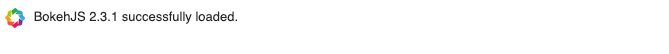1. Line Plot
```nifty_data.plot_bokeh(kind='line') #equivalent to nifty_data.plot_bokeh.line()

```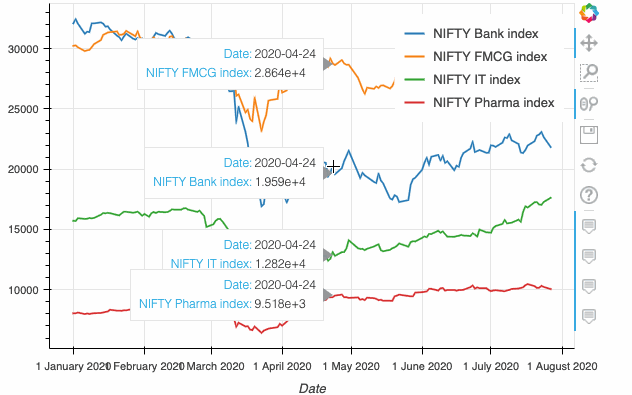Line plot with pandas_bokeh.

1. Scatter Plot
```nifty_data.plot_bokeh.scatter(x='NIFTY FMCG index', y='NIFTY Bank index');

```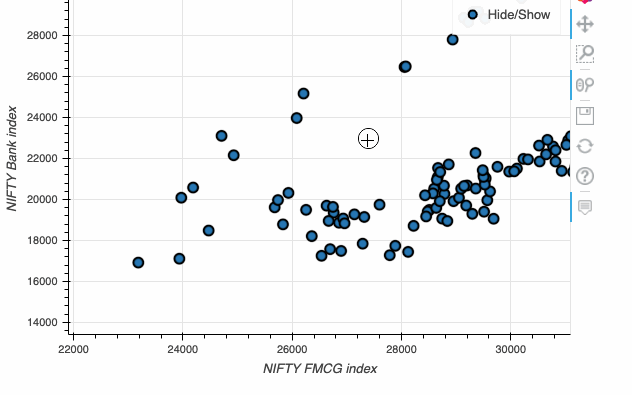Scatter plot with pandas_bokeh.

1. Histograms
```nifty_data[['NIFTY FMCG index','NIFTY Bank index']].plot_bokeh(kind='hist', bins=30);

```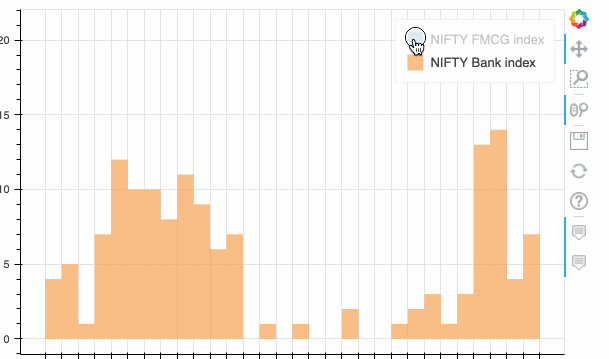Histogram with pandas_bokeh.

1. Bar plots
```nifty_data_resample.plot_bokeh(kind='bar',figsize=(10,6));

```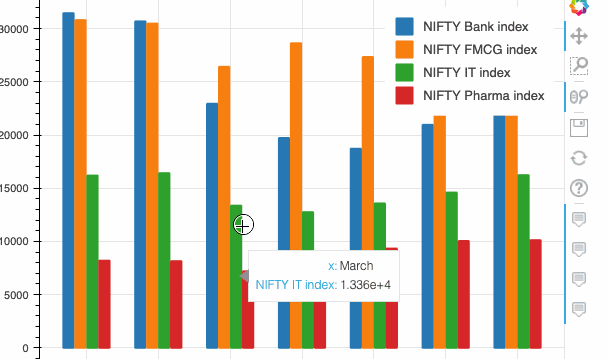Bar plot with pandas_bokeh.

• Stacked bar plots
```nifty_data_resample.plot_bokeh(kind='barh',stacked=True);

```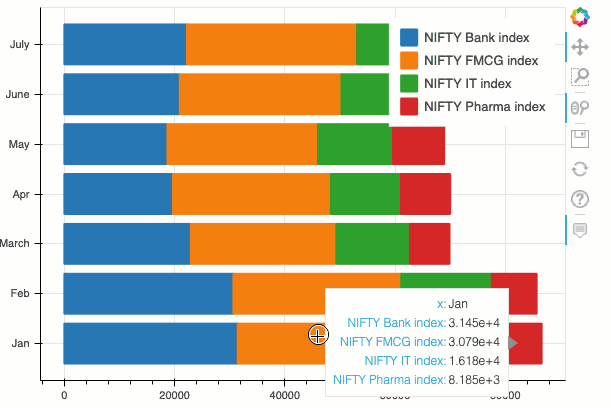Stacked bar plot with pandas_bokeh.

1. Pie Charts
```nifty_data_resample.index=['Jan','Feb','March','Apr','May','June','July']
nifty_data_resample.plot_bokeh.pie(y ='NIFTY Bank index')

```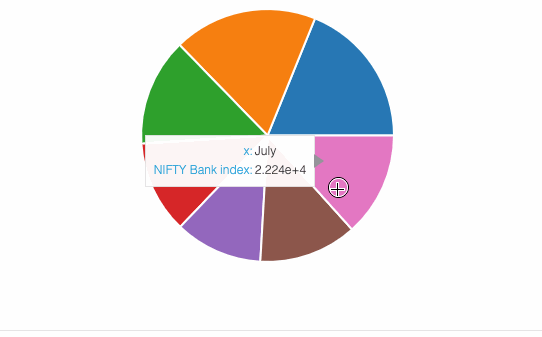Pie chart with pandas_bokeh.

Additionally, you can also create multiple nested pie plots within the same plot:

```nifty_data_resample.plot_bokeh.pie()

```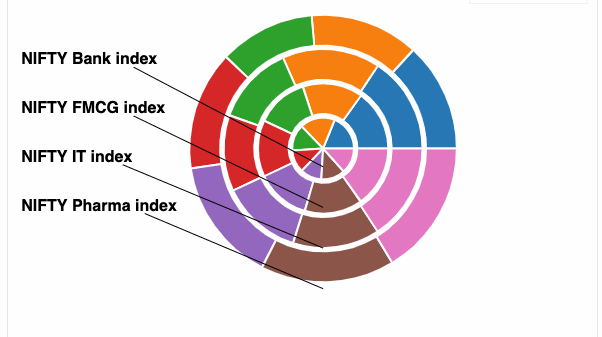Nested pie chart with pandas_bokeh.

This section saw how we could seamlessly create bokeh plots without any significant change in the pandas plotting syntax. Now we can have the best of both worlds without having to learn any new format.

### Plotly Backend for Pandas — plotting with Cufflinks.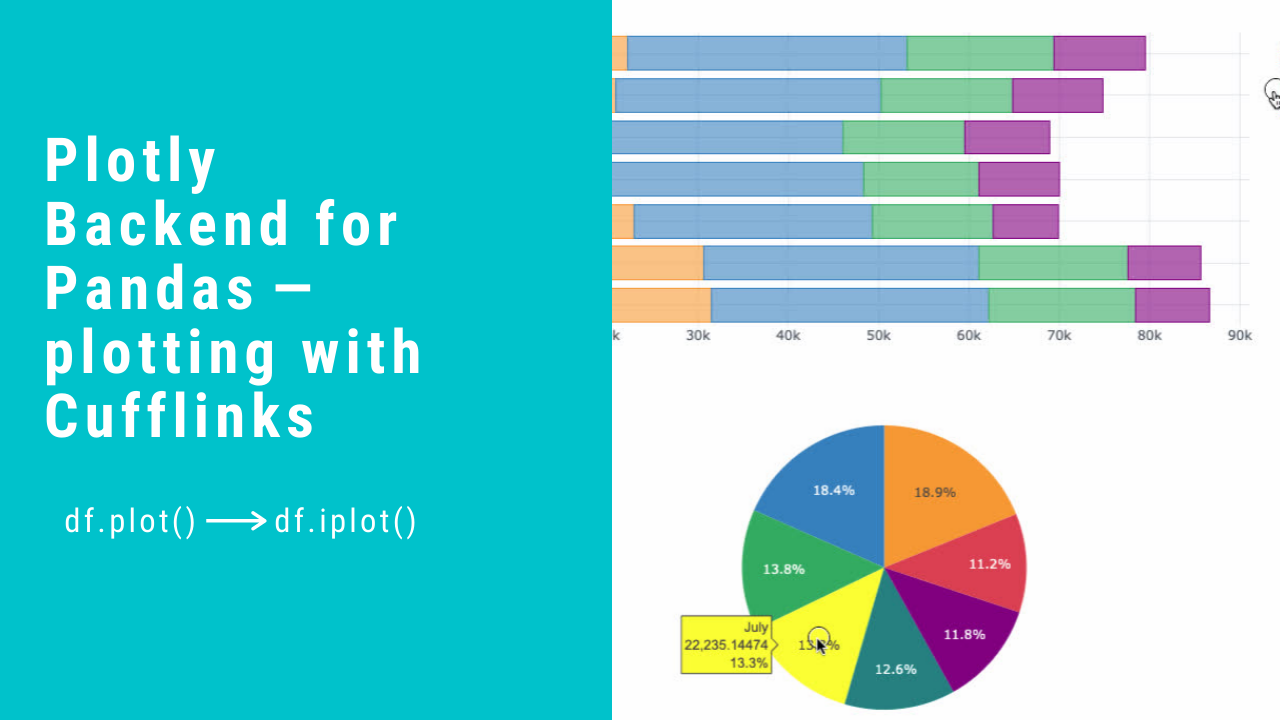Image by Author.

Another commonly used library for data visualization is Plotly. With plotly, you can make interactive charts in Python, R, and JavaScript. As of version 4.8, Plotly came out with a Plotly Express-powered backend for Pandas plotting, which meant that one even does not need to import plotly to create plotly like visualizations.

However, the library I want to mention here is not plotly express, but an independent third-party wrapper library around Plotly called CufflinksThe beauty of cufflinks is that it is more versatile, has more functionalities, and has an API similar to pandas plotting. This means you only need to add a .iplot() method to Pandas dataframes for plotting graphs.

Installation

Make sure you have plotly installed before installing cufflinks. Read this guide for instructions.

```pip install cufflinks

```

Usage

The repository has a lot of useful examples and notebooks to get started.

```import pandas as pd
from IPython.display import display,HTML
#making all charts public and setting a global theme
cf.set_config_file(sharing='public',theme='white',offline=True)

```

That is all. We can now create visualizations with the power of plotly but with the ease of pandas. The only change in the syntax is dataframe.iplot().

1. Line Plot
```nifty_data.iplot(kind='line')

```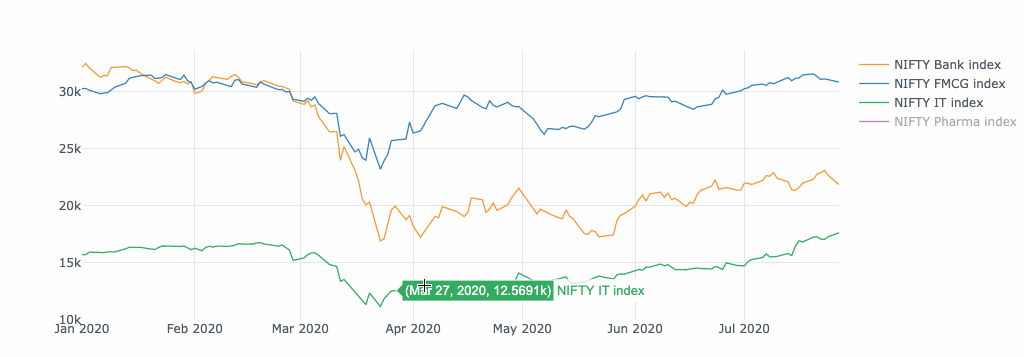1. Scatter Plot

You need to mention the plotting mode for scatter trace while creating a scatterplot. The mode could be lines, markers, text, or a combination of either of them.

```nifty_data.iplot(kind='scatter',x='NIFTY FMCG index', y='NIFTY Bank index',mode='markers');

```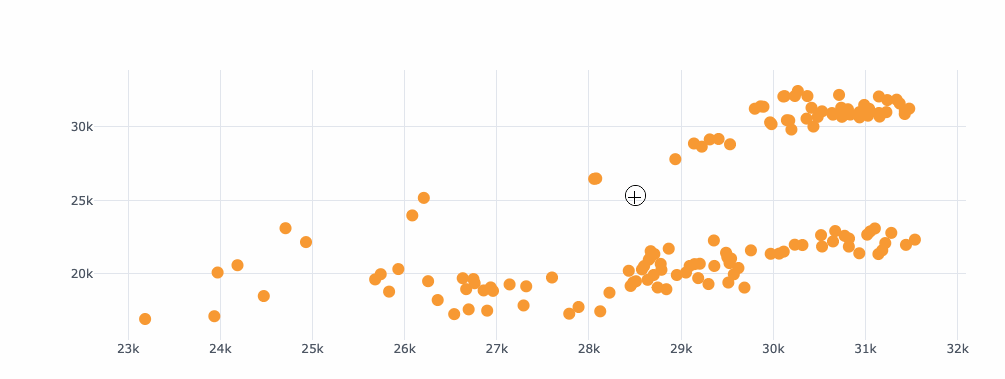1. Histograms
```nifty_data[['NIFTY FMCG index','NIFTY Bank index']].iplot(kind='hist', bins=30);

```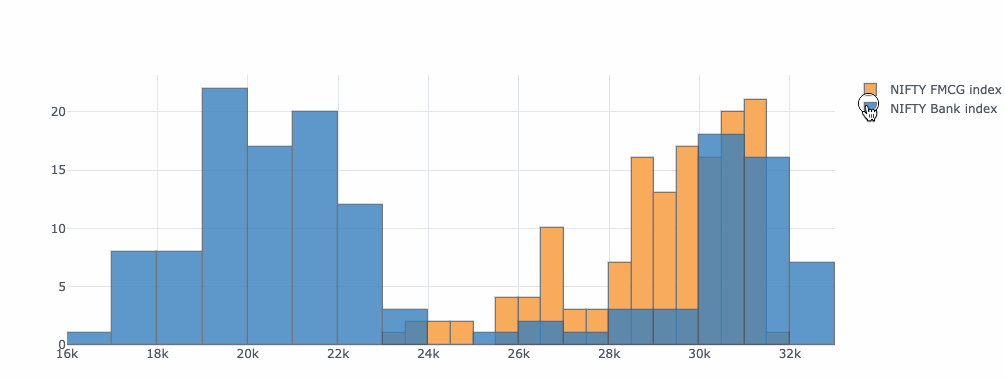1. Bar plots
```nifty_data_resample.iplot(kind='bar');

```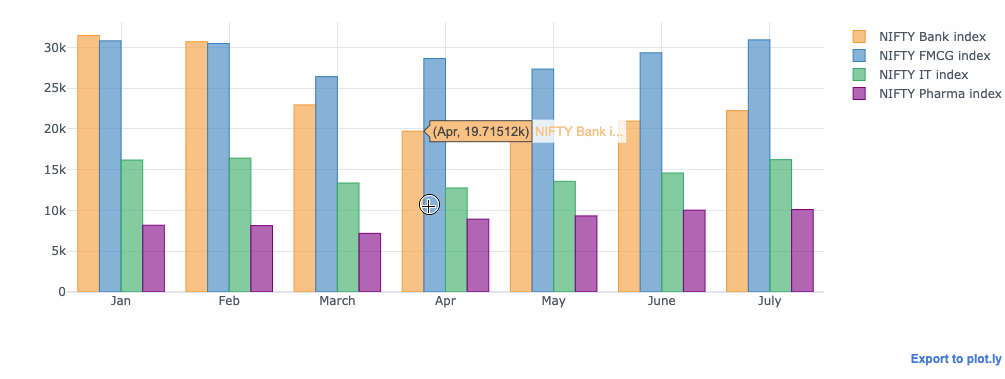• Stacked bar plots
```nifty_data_resample.iplot(kind='barh',barmode = 'stack');

```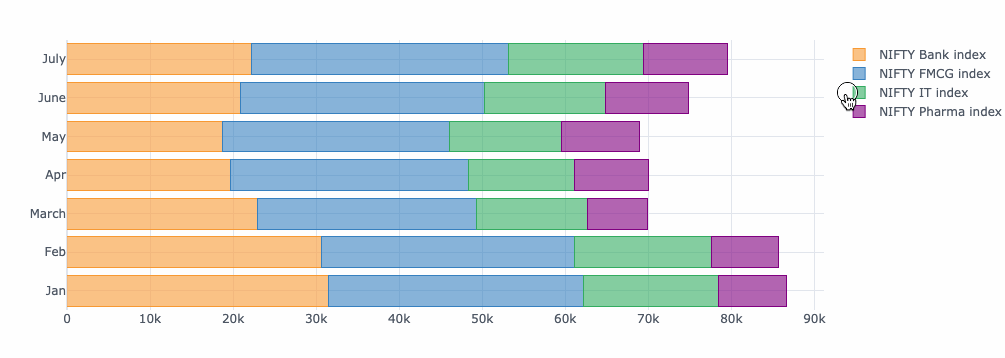1. Pie Charts
```nifty_data_resample.index=['Jan','Feb','March','Apr','May','June','July']
nifty_data_resample.reset_index().iplot(kind='pie',labels='index',values='NIFTY Bank index')

```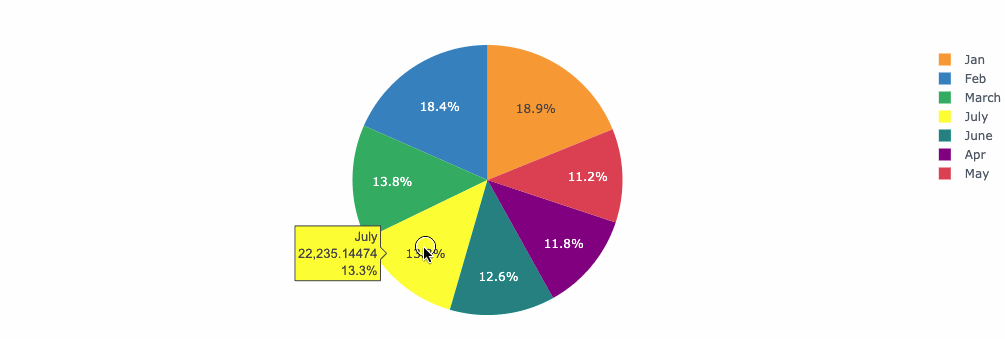The Cufflinks library provides an easy way to get the power of plotly within plotly. The similarity in syntax is another point of advantage.

### Conclusion

The Bokeh or a Plotly plot is self-sufficient in conveying the entire information. Based on your choice and preference, you can choose both or either of them while keeping a focus on the primary purpose of making visualizations more intuitive and interactive at the same time.

Original. Reposted with permission.

Related: